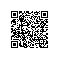# 拜托，面试别再问我最大值最小值了！！！

int find_max(int arr[n]){

int max = -infinite;

for(int i=0; i<n; i++)

if(arr[i]>max)

max=arr[i];

return max;

}

(int, int) find_max_min(int arr[n]){

int max = -infinite;

int min = infinite;

for(int i=0; i<n; i++){

if(arr[i]>max)

max=arr[i];

if(arr[i]<min)

min=arr[i];

}

return (max, min);

}

(1)把大规模拆分成小规模；

(2)小规模分别求解；

(3)小规模求解之后，再综合求解大规模；

(1)将arr[0,n]分为arr[0,n/2]和arr[n/2,n]；

(2)每个子数组分别求解最大值和最小值；

(3)两个子数组的最大值里再取最大值，两个子数组的最小值里再取最小值，就是最终解；

(int, int) find_max_min(int arr[0,n]){

// 递归左半区

(max1, min1) = find_max_min(arr[0, n/2]);

// 递归右半区

(max2, min2) = find_max_min(arr[n/2, n]);

// 再计算两次

max = max1>max2?max1:max2;

min = min1<min2?min1:min2;

return (max, min);

}

(1)入参不是0和n，而是数组的下限和上限；

(2)递归要收敛，当数组的上下限相差1时，只比较一次，直接返回max和min，而不用再次递归；

 (1)递归左半区；

(2)递归右半区；

(3)再进行两次计算；


f(2)=1;【式子A】

f(n)=2*f(n/2)+2;【式子B】

f(n)=1.5n-2;

【式子B】不断展开能得到：

f(n)=2*f(n/2)+2;【式子1】

f(n/2)=2*f(n/4)+2;【式子2】

f(n/4)=2*f(n/8)+2;【式子3】

...

f(n/2^(m-1))=2*f(2^m)+2;【式子m】

f(n)=(2^m)*f(n/2^m)+2^(m+1)-2;【式子C】

• 求最大值，遍历，需要n-1次计算
• 求最大最小值，遍历，需要2n-2次计算
• 求最大最小值，分治，时间复杂度1.5n-2